# Chemistry Topic-Wise Test - 12

## 45 Questions MCQ Test NEET Mock Test Series & Past Year Papers | Chemistry Topic-Wise Test - 12

Description
This mock test of Chemistry Topic-Wise Test - 12 for NEET helps you for every NEET entrance exam. This contains 45 Multiple Choice Questions for NEET Chemistry Topic-Wise Test - 12 (mcq) to study with solutions a complete question bank. The solved questions answers in this Chemistry Topic-Wise Test - 12 quiz give you a good mix of easy questions and tough questions. NEET students definitely take this Chemistry Topic-Wise Test - 12 exercise for a better result in the exam. You can find other Chemistry Topic-Wise Test - 12 extra questions, long questions & short questions for NEET on EduRev as well by searching above.
QUESTION: 1

Solution:
QUESTION: 2

Solution:
QUESTION: 3

### Which of the following statements is correct in relation to the halogenation of alkanes ?

Solution:
QUESTION: 4
Which of the following reactions will lead to the creation of two chiral centers in the product ?
Solution:
QUESTION: 5
trans-2-Buten on reaction with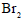in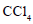forms
Solution:
QUESTION: 6
1-Methylcyclohexene is allowed to react with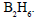The product is then treated with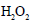and NaOH. The reaction is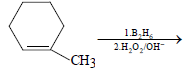The product formed is
Solution:
QUESTION: 7
By which of the following reactions can the conversion of cychlohexene (I) into 1,3-cyclohexadiene (III) be effected
Solution:
QUESTION: 8
Which of the following decolorizes the red-brown of a dilute solution ofin water ?
Solution:
QUESTION: 9
From which of the following hydrocarbons may hydrogen be displaced by a strongly electropositive
metal ?
Solution:
QUESTION: 10
When propyne is treated with aqueous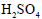,the major product formed is
Solution:
QUESTION: 11
Consider the following reaction sequence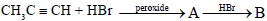The products (A) and (B) are, respectively
Solution:
QUESTION: 12
A hydrocarbon (A) is allowed to react with sodium amide and then with ethyl bromide to produce another hydrocarbon (B) which one ozonolysis produces propanoic acid only. The hydrocarbons (A) and (B) are, respectvely,
Solution:
QUESTION: 13
Acetylene does not react with
Solution:
QUESTION: 14
Which of the following hydrocarbons should be choosen as a starting material to prepare 3-hexanone by the hydration of the former ?
Solution:
QUESTION: 15
Chlorination of toluene in the presence of light and heat followed by treatement with aqueous NaOH and subsequently with dilute HCl gives
Solution:
QUESTION: 16
Among the compounds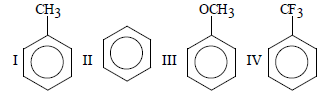the order of decreasing reactivity towards electrophilic substitution is
Solution:
QUESTION: 17
The function of anhydrous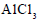in Fridel–Crafts reaction is to
Solution:
QUESTION: 18
The smallest alkane having a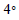carbon is
Solution:
QUESTION: 19
In which of the following reactions is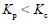?
Solution:
QUESTION: 20
For the equilibrium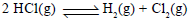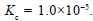If the equilibrium concentration of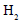and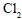is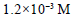and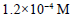respectively then the concentration of HCl is
Solution:
QUESTION: 21
In the reaction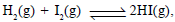the value of the equilibrium constant depends on
Solution:
QUESTION: 22
Consider the equilibrium,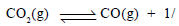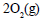The equilibrium constant K is given by (when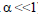)
Solution:
QUESTION: 23
1.7 g of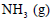is present in a 2-L flask. The active mass ofis
Solution:
QUESTION: 24
For the equilibrium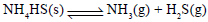Solution:
QUESTION: 25
For the eqilibrium,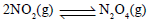+14.6 kcal increase in temperature would
Solution:
QUESTION: 26
When 1 mole of ethanol is mixed with 1 mole of acetic acid, 0.67 mole of ethyl acetate is produced at equilibrium. Calculate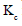for the reaction
Solution:
QUESTION: 27
During thermal dissoication, the observed vapour..........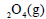is 26.0. Calculate the extent of
dissociation of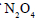(g)
Solution:
QUESTION: 28
When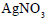is heated in a closed vessel,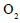gas is liberated and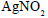is left behind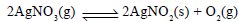,At equilibrium
Solution:
QUESTION: 29
For the heterogeneous equilibrium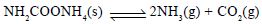the value of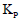is
Solution:
QUESTION: 30
The pH of a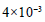M HCl solution is
Solution:
QUESTION: 31

The pH of a solution formed by maixing 40.0 mL of 0.1 M HCl with 10.0 mL of NaOH is

Solution:

40ml  of 0.1MHCl
(0.1/1000)×40
= 0.004 moles of HCl
Similarly, 10ml of 0.45MNaOH
= (0.45)/1000 × 10
= 0.0045 moles of NaOH
Thus, NaOH left unneutralised = 0.0005 moles in a volume of 50ml
Concentration of NaOH = (1000×0.0005)/50=0.01
= 10-2M
Therefore, [OH-]=10-2M or [H+]
= 10-12M or pH = 12.0

QUESTION: 32
The solubility of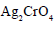is
Solution:
QUESTION: 33
The solubility product of CuS,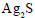and HgS are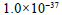,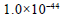and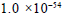respectively. The
solubilities of these suophides are in the order
Solution:
QUESTION: 34
When equal volumes of the following solutions are mixed, the precipitation of AgCl will occur only with (given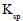of Agcl=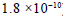)
Solution:
QUESTION: 35
For a weak monoprotic acid, the pH of a given concentration is calculated by the equation
Solution:
QUESTION: 36
For a salt of a weak acid and a weak base
Solution:
QUESTION: 37
The solubility product of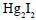is
Solution:
QUESTION: 38
Which of the following is arranged in order of increasing density ?
Solution:
QUESTION: 39
Which of the following alkali-metal hydroxides is the least soluble in water ?
Solution:
QUESTION: 40
Glauber’s salt is represented by the formula
Solution:
QUESTION: 41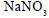being heated to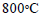gives
Solution:
QUESTION: 42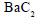reacts with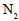to produce
Solution:
QUESTION: 43
Milk of lime is
Solution:
QUESTION: 44
Which of the following is arranged in order of decreasing solubility in water ?
Solution:
QUESTION: 45
Which of the following compounds is a perioxide
Solution: